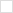TWiki>P1076 Web>CollectedRequirements>VectorLiteralntrospection (revision 8)EditAttach

# Type Introspection on Vector Literals

## Proposal Information

• Date Proposed: 2011-07-15
• Date Last Updated: 2011-07-15
• Priority:
• Complexity:
• Focus: General Language, but specifically Testbench

## Requirement Summary

Do introspection on vector (single dimensional array) literal values

## Rationale

Vectors can be used to create an "argv" like set of values to an interface (such as a subprogram). To effectively use this capability within an overloaded subprogram environment, we need introspection of vector literals

## Use Models

### Use Model: Simplify use of aggregates in subprogram calls

#### Current Situation

Consider the following two subprogram declarations:

```function to_integer_vector (BV : in boolean_vector ) return integer_vector ;
function to_integer_vector (SLV : in std_logic_vector ) return integer_vector ;```

Calling the subprograms with an aggregate results in an ambiguous expression. Note since they return a variable number of values, generally they are used within the call to another subprogram.

`--! CrossCovBin1.ICover(to_integer_vector( ((Empty='1'),(Rdy='1')) )); -- ambiguous`

One way to address this is to use a type qualifier.

`CrossCovBin1.ICover(to_integer_vector( boolean_vector'((Empty='1'),(Rdy='1')) )); `

Ironically, the language already does introspection of vectors when concatenation is used instead of aggregates.

`CrossCovBin1.ICover(to_integer_vector( ((Empty='1') & (Rdy='1')) )); -- not ambiguous`

#### Code Simplification with Proposal

With proposal, type qualifiers are not required with aggregates

`CrossCovBin1.ICover(to_integer_vector( ((Empty='1'),(Rdy='1')) )); -- enhanced support`

### Use Model: Simplify use of string literals?

It would be nice if this solution also addressed string literals. If this proposal were to also include string literals, then the following would no longer be ambiguous. However, the main intent here is to solve the aggregate issue and this issue in particular was addressed in VHDL-2008 with std.textio.swrite.

`std.textio.write(buf, "This does not contain std_ulogic values") ; `

### Use Model: More effectively overload subprograms

#### Current Situation (from randomization package):

In the my randomization package there are two flavors of creating discrete distributions. The first, DistInt, takes in a vector of weights and returns a value that is between 0 and N (where N = Weight'length-1). Where each weight will occur 100*(weight/Sumof(weights)) percent of the time. The second, DistValInt, takes in a vector of value and weight pairs. When a particular weight is selected, the corresponding value is returned.

```    impure function DistInt ( Weight : integer_vector ) return integer ;
impure function DistValInt ( A : DistType ) return integer ;```

The following shows two calls to these function methods.

```    TestData1   := RV.DistInt ( (70, 10, 10, 5, 5) ) ;
TestData2  :=  RV.DistValInt ( ((1,70), (5,10), (9,10), (13, 5), (17,5)) ) ;```

#### Current Situation - Alternative Implementation:

To facilitate remembering the name of the function required for weighted distribution, it would be easier if each were named DistInt

. This is shown below.

```    impure function DistInt ( Weight : integer_vector ) return integer ;
impure function DistInt ( A : DistType ) return integer ;```

Unfortunately, the function method calls now require type qualifiers:

```    TestData1   := RV.DistInt ( integer_vector'(70, 10, 10, 5, 5) ) ;
TestData2  :=  RV.DistInt ( DistType'((1,70), (5,10), (9,10), (13, 5), (17,5)) ) ;```

#### Code Simplification with Proposal

The enhancements proposed, gives us the advantage of allowing both functions to have the same name and allows the call to be able to be done without type qualifiers. Function Declarations:

```    impure function DistInt ( Weight : integer_vector ) return integer ;
impure function DistInt ( A : DistType ) return integer ;```

Method calls without type qualifiers due to enhancements:

```    TestData1   := RV.DistInt ( (70, 10, 10, 5, 5) ) ;
TestData2  :=  RV.DistInt ( ((1,70), (5,10), (9,10), (13, 5), (17,5)) ) ;```

## Arguments FOR

• User-code simplification -- BAH

## Arguments AGAINST

• Compiler complexity?